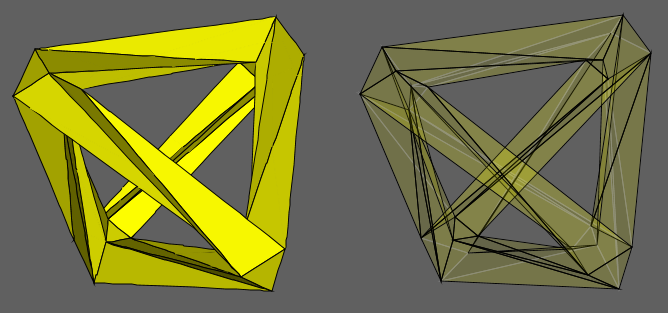Acronym ... Name hyperbolic order 7 triangular tiling `©` Circumradius sqrt[(1-cos2(π/7))/(3-4 cos2(π/7))] = 0.873057 i Vertex figure  Dual o3o7x Confer general polytopal classes: regular   noble polytopes Externallinks©

There exists a regular modwrap of this tiling, obtained by identifying every 8th vertex on either Petrie polygon. The resulting figure {3, 7}8 then also results in choosing N=4 and has genus 3.

Incidence matrix according to Dynkin symbol

```x3o7o   (N → ∞)

. . . | 6N |   7 |   7
------+----+-----+----
x . . |  2 | 21N |   2
------+----+-----+----
x3o . |  3 |   3 | 14N
```